# SAS/STAT Examples

## Bayesian Binomial Model with Power Prior Using the MCMC Procedure

Contents | SAS Program | PDF

## Overview

Power priors (Ibrahim and Chen; 2000 ) are a useful family of informative priors when relevant historical data is available. You can use power priors when you want to take historical data into account while analyzing similar, current data. This example illustrates fitting a Bayesian binomial model with a power prior and also demonstrates two useful features of PROC MCMC: using nonstandard distributions and assigning different likelihood functions to different subsets of the data.

## Analysis

Suppose that, in addition to data from a current study, you have similar data from a historical data set or pilot study. A power prior enables you to capture the information collected in the pilot study and incorporate it into analysis and inference in the current study. The historical data gets integrated via an informative prior and provides a convenient way of updating past knowledge.

A power prior can be expressed as a product of the weighted likelihood ofconditional on the historical data and a prior distribution on the parameters before any data is observed. More formally, the power prior distribution ofis written as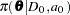where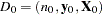are the historical data (from the pilot study),is the likelihood ofconditional on historical data,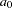is a discounting or scale parameter withthat controls the amount of weight you want to put on the historical data, andis a prior forbefore the historical data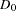are observed.

The posterior distribution ofis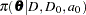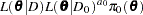( 1 )

whererepresents the data from the current study,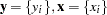for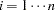,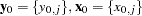forrepresents the historical data, andrepresents the density function for a single observation in either the historical or the current data set.

You can combine the two data sets to form a new data set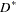and rewrite the posterior distribution in Equation 1 as the following:( 2 )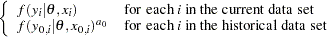The posterior distribution Equation 2 is used to model the hypothetical clinical trial setting described in this example. The following SAS statements create the PTRIALS data set that represents binomial data collected in a pilot study of a clinical trial.

```      data ptrials;
input y n;
datalines;
5 163
;
```

In the INPUT statement,represents the number of successes out ofindependent Bernoulli trials. Similarly, for the current study, suppose you have the following hypothetical clinical trials data set BTRIALS .

```      data btrials;
input y n @@;
datalines;
2  86
2  69
1  71
1 113
1 103
;
```

In order to fit a model with a power prior, first combine both the current and pilot study data sets. The indicator GROUP variable takes on the values ' CURRENT ' and ' PILOT ' for the current and pilot study data set, respectively. The following SAS statements demonstrate how to create a new combined data set ALLDATA .

```      data alldata;
set btrials(in=i) ptrials;
if i then group='current';
else group = 'pilot';
run;
```

Suppose you want to fit a Bayesian binomial model with a power prior for a hypothetical clinical trial setting with density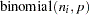( 3 )

where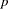is the success probability forobservations.

The likelihood function for each of the observations is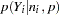( 4 )

wheredenotes a conditional probability mass function. The binomial density is evaluated at the specified value ofand corresponding success probability. Then, a power prior is used to assign each observation in the combined data set a binomial or weighted binomial likelihood function depending whether the observation was from the current or pilot study, respectively.

Suppose the following power prior is placed on the success probability parameters, whererepresents the binomial likelihood: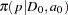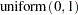( 5 )

The variablesand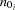represent the historical successes and sample size, respectively, andindicates a prior distribution. Suppose for this example that the discounting factor is set to. The diffuseprior expresses your lack of knowledge about the success probability.

According to Bayes’ theorem, the likelihood function and prior distribution determine the posterior distribution ofas given in Equation 2 . PROC MCMC obtains samples from the desired posterior distribution, which is determined by the specified prior and likelihood. It does not require the exact form of the posterior distribution.

The following SAS statements use the likelihood and power prior distribution to fit the Bayesian binomial model with the historical data. The PROC MCMC statement invokes the procedure and specifies the input data set. The SEED= option specifies a seed for the random number generator, which guarantees the reproducibility of the random stream. The NMC= option specifies the number of posterior simulation iterations.

```   ods graphics on;
proc mcmc data=alldata seed=1181 nmc=10000;
parms p 0.2;
begincnst;
a0 = 0.5;
endcnst;
prior p ~ uniform(0,1);
llike = logpdf('binomial',y,p,n);
if (group='pilot') then llike = a0*llike;
model general(llike);
run;
ods graphics off;
```

The PARMS statement designatesas a parameter in the model and assigns an initial value of 0.2 to it. The programming statements enclosed by the BEGINCNST and ENDCNST statements are executed once per procedure call and designate the discount factor for the power prior.

The PRIOR statement specifies a prioras given in Equation 5 . The LLIKE= assignment statement assigns the log-likelihood function for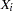as given in Equation 3 ; the LOGPDF function calculates the logarithm of the binomial function. The IF/THEN statement uses the GROUP indicator variable to weight the binomial likelihood when the observation is from the pilot study.

The MODEL statement uses the GENERAL function, which indicates that you are using a SAS statement to construct a nonstandard density or distribution. The argument is an expression that takes the value of the logarithm function of the prior or likelihood distribution. In this case, the nonstandard density is the binomial or weighted binomial likelihood, and the argument is the logarithm of the density.

Figure 1 displays convergence diagnostic plots forto assess whether the Markov chains have converged. Inferences should not be made if the Markov chain has not converged.

Figure 1 Bayesian Binomial Model Diagnostic Plots forThe trace plot shows that the mean of the Markov chain is constant over the graph and is stabilized. The chain is able to traverse the support of the target distribution, and the mixing is good. The trace plot shows that the Markov chain appears to have reached a stationary distribution.

The autocorrelation plot indicates low autocorrelation and efficient sampling. The kernel density plot shows a smooth, unimodal posterior marginal distribution for the parameter.

PROC MCMC produces formal diagnostic tests by default, but they are omitted here because informal checks on the chains, autocorrelation, and posterior density plots show desired stabilization and convergence.

Figure 2 reports summary and interval statistics for the parameter’s posterior distribution.

Figure 2 Posterior Model Summary of Binomial Model
The MCMC Procedure

Posterior Summaries
Parameter N Mean Standard
Deviation
Percentiles
25% 50% 75%
p 10000 0.0200 0.00602 0.0156 0.0194 0.0237

Posterior Intervals
Parameter Alpha Equal-Tail Interval HPD Interval
p 0.050 0.0100 0.0333 0.00900 0.0316

Using the power prior, the posterior mean of the success probability is 2.00% with a 95% credible interval of, providing evidence of a treatment effect.

Modeling the clinical trial data set in a Bayesian binomial model with a power prior is a good way to make use of the pilot study. It enables you to use a different class of informative priors and incorporates relevant historical data and information.

## References

Ibrahim, J. G. and Chen, M.-H. (2000), “Power Prior Distributions for Regression Models,” Statistical Science , 15, 46–60.